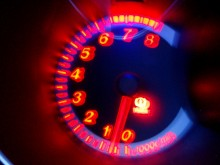How To Convert Rpm To M/s?"I think it would be helpful to have more information as to what you are trying to achieve here, because these two measurements don't really fit together in a straightforward way, so it really depends what the situation is that you are looking at, before we can establish the correct calculation. One thing that is clear, is that the diameter of the article that is rotating, is very important because this size will affect the result. For example you can calculate the miles per hour figure in a car by taking the revolutions per minute and multiplying by the wheel diameter and then dividing the result by the gear ratio multiplied by 336 (or the calculation (Revolutions Per Minute * wheel diameter) / (Gear Ratio * 336)). From this you can calculate meters per second, by multiplying the miles per hour figure by 0.447. Obviously if your Revolutions Per Minute calculation is not in a car, then the GearRatio figure will not be relevant but there will most likely be something that needs to be taken into account which restricts the speed in the same way as the gear, causing friction and resistance. Of course, the term RPM is used in many different scenarios and a car is just one, so this calculation may not make sense entirely in other contexts, for example, a vinyl record player also uses revolutions per minute to describe the speed of either an LP or single."
thanked the writer.
4000 rpm to m/s ?
thanked the writer.
Como converter 120 rpm em m/s
thanked the writer.
Convert rpm to m/s
thanked the writer.
Shaft rotates at 3600rpm.
A. What is its angular velocity in rad/s?
B. How many radians does it turn through in 1.5 seconds?

We know that there are 2p radians for every revolution and 60s for every minute, so

Once we know the velocity, part b is easy.

Problem Two: A windmill has 40m long radius blades which rotate at 30 rpm. What is the linear velocity of the blade's tips?

First we need to convert rpm to rad/s, because the equations used to convert rotational to linear movement have to have rad/s.

30rpm * 2rad ÷ 60s = 3.142rad/s

Then we just use the equation v = r * to convert to linear velocity.

V = 40m * 3.142rad/s
v = 125.68m/s
thanked the writer.